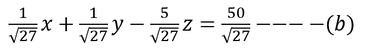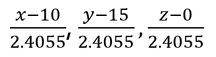top of page
##### 2. Warping Factor Calculation

1. Warping Angle Calc

## Warping Angle Calculation Example of a Quadrilateral Element:

• Let "ABCD" is the quadrilateral element with the nodal co-ordinates A(10,15,0), B(25,25,0), C(35,15,5), and D(25,5,-5) as shown in the figure below.• With ABCD quadrilateral element two sets of triangular planes are possible ABD &BCD and ABC & ACD.

Step-2: Angle Between ABD & BCD:

• First Construct two Triangular planes ABD (Plane-1) & BCD (Plane-2) as shown in the figure above.

• Now let us write equations of the first set of planes shown below.

Equation of the plane-1, ABD is:Equation of the plane-2, BCD is:Normal Form of Equations of Plane (1) & (2):The Direction Cosines of Plane-1 & Plane-2 are:So, The angle between two planes,Angle between Plane-1 &2 is (θ1 )=42.998 degree

Step-2: Angle Between ABC & ACD:

• Construct two Triangular planes ABC (Plane-1) & ACD (Plane-2) as shown in the figure below.

• Now let us write equations of the first set of planes shown below.Equation of the plane-3, ABC is:Equation of the plane-4, ACD is:Normal Form of Equations of Plane (3) & (4):The Direction Cosines of Plane-3 & Plane-4 are:So, The angle between two planes,Angle between Plane-3 &4 is (θ2 )=50.860 degree

From Step-1 & Step-2: The Warping Angle is maximum of the two angle calculated

Warping Angle = Max(θ1, θ2)=50.86°

2. Warping Angle Calc
##### 2. Warping Factor Calculation Example of a Quadrilateral element
• Let "ABCD" is the quadrilateral element with the nodal co-ordinates A(10,15,0), B(25,25,0), C(35,15,5), and D(25,5,-5) as shown in the figure below.Direction Cosines of AC :

Direction Cosines of BD :• Now, We have to find the common normal to AC & BD.

• Let, The direction cosines of Common normal is l,m &n.

Since, AC ⊥ Common NormalSimilarly, Since, BD⊥Common NormalSo, the direction cosines of common Normal are:Equation of a plane Passing Through A&C, whose normal is perpendicular to AC is:Since it is passing through A(10,15,0)

So, Equation of Plane Passing through AC is:Similarly, Equation of a plane Passing Through B&D, whose normal is perpendicular to BD is:Since it is passing through B(25,25,0) ⟹ p=50

So, Equation of Plane Passing through BD is:Now, let's find out the perpendicular distance between nodal points to the Average Plane.

The Perpendicular distance of Plane (b) from point-A(10,15,0) is:Perpendicular distance of Average plane from Point-A is, AA'=4.811/2=2.4055 (=h)

Similarly, BB′′=CC′′=DD′′= 4.811 and BB′=CC′=DD′=2.4055

A’B’C’D’ is the Average normal plane of the Quad Element ABCD, which is equi-distance from all Nodes of Quad Element

Find the Projection of Nodes on the Average Normal Plane:

Projection of Node “A” on average normal plane is A’.

Since, Plane A’B’C’D’ is parallel to Plane-a & Plane-b.

So, the direction cosines of Plane A’B’C’D’ are as follows:Let us assume, the projection of Node-A on Average Normal plane is A′ (x,y,z).

So, the direction cosines of AA′  are,So, the direction cosines of line AA′ are ⇒By equating these values with l, m, n, we will get x, y and z values.So, the projection of Node-A is, A’(10.463,15.463,-2.315)

Similarly, the Projections of other nodes B, C and D are,

B′(24.537,24.537,2.315), C′(35.463,15.463,2.685), D’(19.537,4.537,-2.685)

Area of the projected Quadrilateral on Average Normal Plane A'B'C'D':

Area of the Quad Element,∆A′B′C′ D′=∆A′B′C′+∆A′C′D′

A’B’=17.374, B’C’=14.207, C’D’=20.046, D’A’=14.207, A’C’=25.495

S1=(Perimeter of A′B′C′)/2=28.538,

S2=(Perimeter of A′C′D′)/2=29.874

Area, A=√(S(S-a)(S-b)(S-c))

Area of the projected quadrilateral on average normal plane, A=A1+A2=117.8757+141.9319=259.8

So, the Warping factor is the ratio between "distance between two parallel planes and Square root of the area of the projected quadrilateral on average normal plane.

Warping Factor= 2h/√A= (4.811)/√(259.8) = 0.298bottom of page